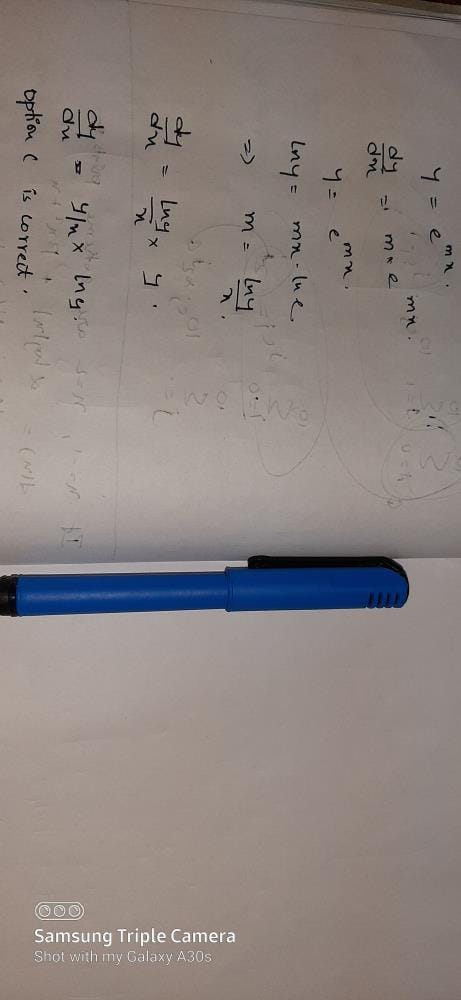The differential equation with respect to the...

### Related TestThe differential equation with respect to the curve y=emx is
• a)
(dy/dx)=(y/x)logx
• b)
(dy/dx)=(x/y)logy
• c)
(dy/dx)=(y/x)logy
• d)
(dy/dx)=(x/y)logxKhaja MoinuddinView courses related to this question Explore JEE courses
 Explore JEE coursesView courses related to this question1 Crore+ students have signed up on EduRev. Have you?

• ### Let f : R→ Rbe a differentiable function with f(0) =0. If y = f(x) sat... more(Scan QR code)